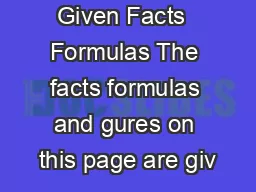# SAT Math Given Facts Formulas The facts formulas and gures on this page are giv - PDF document#### SAT Math Given Facts Formulas The facts formulas and gures on this page are giv - Description

30 60 45 45 A bh c Special Right Triangles A 960r C 2 960r A lw V lwh V 960r The number of degrees of arc in a circle is 360 The sum of the measures in degrees of the angles of a triangle i s 180 wwweriktheredcomtutor pg 1 SAT Math Given Facts ID: 2533 Download Pdf